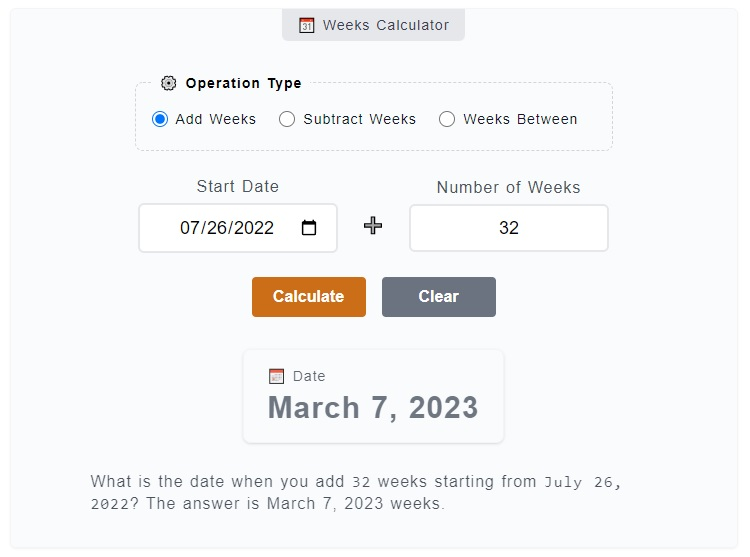# Weeks Calculator

The Weeks Calculator is used to get the number of weeks between two dates, add and subtract weeks from a starting date. For example, you want to know how many weeks are there in between and ? Just enter them on the calculator. You can also add `7 weeks` or subtract `14` weeks using this calculator.

📆 Weeks Calculator
⚙️ Operation Type

### 🌞 Number of Weeks

21 weeks

How many weeks are there between `June 23, 2023` and `November 17, 2023`? There is a total of `21` weeks.

## How to Use the Weeks Calculator

To use the Weeks Calculator, you just need to pick first the operation you want to use - add weeks, subtract weeks or get the weeks between two dates. You just need to enter the values on corresponding inputs and click on `Calculate` button. Here's a more detailed step by step guide.1. Step 1

Above the main calculator, you can find the `⚙️ Operation Type` field set. It is where you need to choose from the operation options - add weeks, subtract weeks and get the weeks between. For this example, let's select the `weeks between` option which is already selected by default.

2. Step 2

After you have chosen an operation type, the next thing to do is to fill the input fields - `Start Date` and `End Date`. The Start Date represents the base or reference date of the count-off while the End Date is where the count-off ends.

3. Step 3

The final step is to click on the `Calculate` button to get your results. You can reset the filled input fields as well by just clicking on the `Clear` button.

In case you are doing some addition or subtraction of weeks from the starting date, first select the `add` or `subtract` as the type of operation. Then, enter the starting date and the number of days want to add or subtract on their corresponding fields.

Although we have a much specific calculator for weeks from now (➕ add weeks) and weeks ago (➖ subtract weeks), this calculator has more option to change the starting date and combined with weeks between two dates operation.